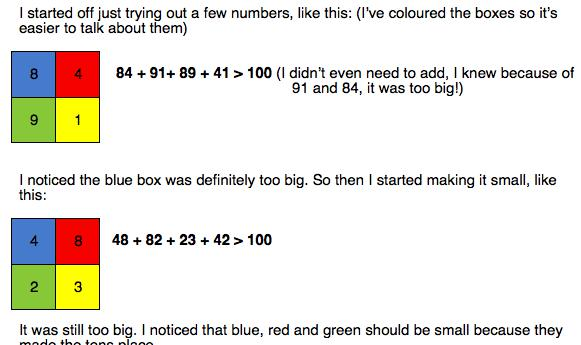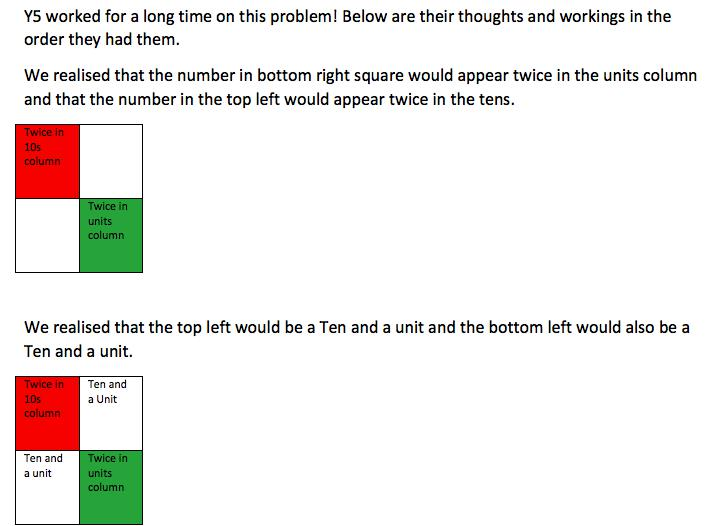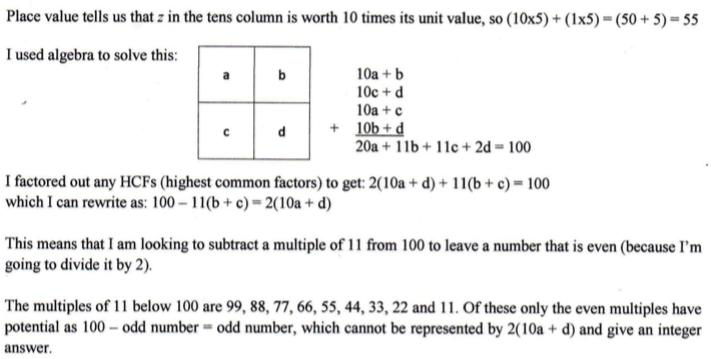#### You may also like### Pebbles

Place four pebbles on the sand in the form of a square. Keep adding as few pebbles as necessary to double the area. How many extra pebbles are added each time?### Bracelets

Investigate the different shaped bracelets you could make from 18 different spherical beads. How do they compare if you use 24 beads?### Sweets in a Box

How many different shaped boxes can you design for 36 sweets in one layer? Can you arrange the sweets so that no sweets of the same colour are next to each other in any direction?

# Reach 100

##### Age 7 to 11Challenge Level

We had nearly 100 submissions for this challenge.  Thank you to everyone who sent in their ideas.

Tipu who learns at home in India wrote :

My mother helped me type up what I did and thought.

Here is a glimpse of what arrivedand I recommend that you look at the whole document here: Tipu.docx

Well done, Tipu, you have described carefully what you did and given clear reasons for your thinking.

Alex, Jocelyn and Mackenzie from the Maths Club at South Walney Junior School wrote:

To start with, we realised that the bigger numbers 85/94 make a bigger target number. From now on, we chose smaller numbers.
We then started to look at 1/2/3/4 and tried different switching arrangements - this did not work. We noticed that the bottom right digit in the grid was used twice. So we started using the number 9 - this didn't work.
Then, systematically we tried 8. We put the 8 in its position, we doubled this digit to make 16, then added 3 + 1 to make a multiple of 10. We did this because we realised that in the column addition, the units digit must end with a zero! When we put these numbers into their grid position we worked out the 4th missing digit - which was 2!.
So our solution was 21, 38, 23 & 18.
We also found a solution for 7 in the bottom right grid using the same strategy. Our solution is 14, 27, 12 & 47.
We couldn't find a solution for the other numbers - but this raised the question 'What was so special about the numbers 7 & 8???' We are still looking for an answer to this...
. . . . .

Y5 at Rickmansworth Park JMI also responded to this challenge.
Here is the start of their work and below you can download the whole document that is well worth a read.The full document can be viewed here: Y5.docx

Kriti from Bootham Junior School wrote:

Imagine the letters A, B, C, and D stand for 4 numbers.
(A x 10) + B   +   (C x 10) + D   +   (A x 10) + C   +   (B x 10) + D:  (20 x A) + (11 x B) + (11 x C) + (2 x D) = 100
The eleven times table is:
1 x 11 = 11  can be eliminated because (B + C) is at least 3
2 x 11 = 22  can be eliminated for the same reason
3 x 11 = 33  can be eliminated because 67 is an odd number and (20 x A) + (2 x  D) is an even number
4 x 11 = 44
5 x 11 = 55  can be eliminated for the same reason
6 x 11 = 66
7 x 11 = 77  can be eliminated for the same reason
8 x 11 = 88  can be eliminated because (20 x A) + (2 x D) is at least 24
9 x 11 = 99  can be eliminated for the same reason

A = 2, B = 3, C = 1, and D = 8,
A = 1, B = 2, C = 4, and D = 7,    are the only solutions.      ARE THEY?

Emad, Omar, Mehak, Nihal and Kishaaragan from Northgate Primary (Crawley) wrote:

First we tried making the units add up to ten so that there was a place holder of zero in the units. After lots of attempts we found this method wasn't working, so we tried making the units add up to 20. Because this meant you had to carry the 2 across to the tens column, the tens column had to add up to 8. When you do this you have a zero in the units and in the tens. This means you can make 100. If the units value is less or more than 20 there will no possibility of reaching 100. This made our working out quicker because we were able to stop the calculations when we knew they wouldn't work. We also tried making solutions with zero to see what happened, this helped us find lots more answers. Here are some of the solutions we found: 21 and 38, 14 and 27, 29 and 30, 23 and 18, 20 and 48, 19 and 27, 16 and 07.

Just to remind you - you had to use the digits 1 through to 9 so zero was not allowed, but if we did allow zero, 16 and 07 work.

Zach sent in a pdf which is a wonderfully clear account of how he tackled the problem.  You can see the start of it below and go here to read it all:  Zach.pdfPaz from King´s College, The British School of Alicante in Spain, sent in the following:

I worked on this puzzle with six other students in Year 6: Enrique, Pablo, Ivan, José Luis, Aldo and Archie. We decided to try lots of combinations and explain to each other what we noticed to help us find the answers.

If our four boxes are:

 a b c d

We realised that a, b and c must be quite small otherwise the total quickly went above 100 when we did:
a b; + a c; + b d; + c d

We also realised that d is quite important because we add that twice in the units column. We looked carefully at how to make b + c + 2d end in a zero.

I found one answer: a = 1; b = 4; c = 2; d = 7

And we realised we could rearrange (transpose) this: a = 1; b = 2; c = 4; d = 7

Ivan also found a different pair of answers: a = 2; b = 3; c = 1; d = 8
Or: a = 2; b = 1; c = 3; d = 8

But we can't be sure that these are all of the answers. We looked for more but we couldn ´t find any others.

4/6 MEP team from Lumen Christi Primary School, Point Cook in Australia sent in another excellent document that I recommend you read. You can access it here: .doc  or .pdf
Just before the end of 2015 we had some good ideas from Year 4, at Kowloon Junior School, Hong Kong you can see Here.doc

There certainly was a great response to this challenge, I'm sorry we have limited space and cannot show more. Thank you all so much and well done for explaining your thoughts and observations so clearly.